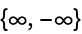#Function Repository Resource:

# MinMaxRounded

Get the minimum and maximum of a list rounded to a multiple

Contributed by: Sander Huisman  |  SHuisman
 ResourceFunction["MinMaxRounded"][list] gives the minimum and maximum of list, rounded down and up, respectively. ResourceFunction["MinMaxRounded"][list,a] gives the minimum and maximum of list, rounded down and up to a multiple of a. ResourceFunction["MinMaxRounded"][list,a,{f,g}] gives {f[Min[list],a],g[Max[list],a]}.

## Details and Options

ResourceFunction["MinMaxRounded"] is used to find nice, readable numbers for the range of the values of a list.
ResourceFunction["MinMaxRounded"][list] gives {Floor[Min[list]],Ceiling[Max[list]]}.
ResourceFunction["MinMaxRounded"][list,a] gives {Floor[Min[list],a],Ceiling[Max[list],a]}.
Common values for {f,g} are:
 {Floor,Ceiling} minimum and maximum values are rounded "outward" {Ceiling,Floor} minimum and maximum values are rounded "inward" {Round,Round} minimum and maximum values are rounded to the closest value

## Examples

### Basic Examples (2)

Find the rounded-down minimum and rounded-up maximum for a list of values:

 In:=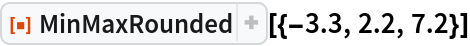Out=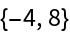Find the minimum rounded down to a multiple of 5 and the maximum rounded up to a multiple of 5 for a list of values:

 In:=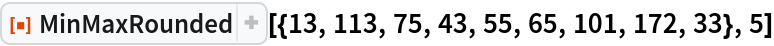Out=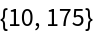### Scope (2)

By default, the minimum is rounded down and the maximum rounded up:

 In:=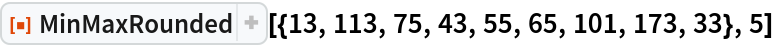Out=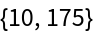Round the minimum up and the maximum down:

 In:=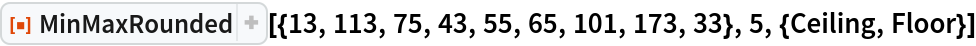Out=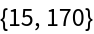Round the minimum and the maximum to the closest multiple of 5:

 In:=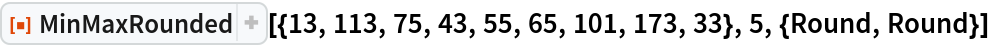Out=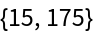If the minimum is positive, return 0 and do not round the maximum:

 In:=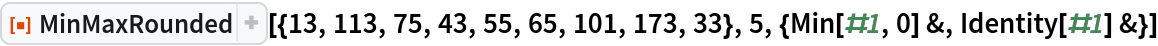Out=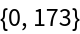### Applications (1)

Find a "nice" plot range that includes all the data:

 In:=Out=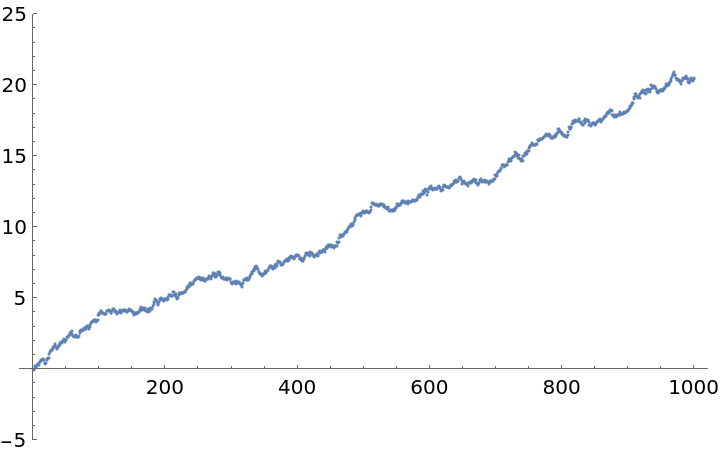### Possible Issues (1)

An empty list returns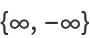:

 In:=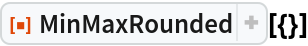Out=